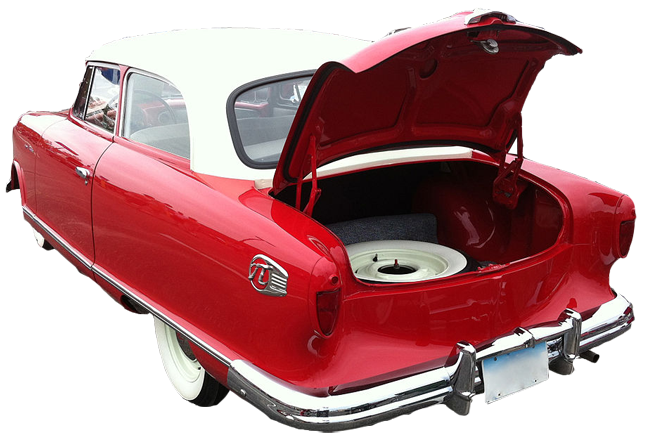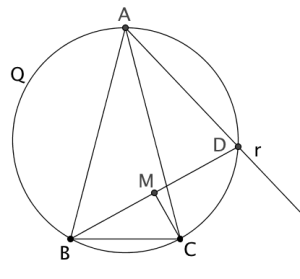This weekend, the team is wishing a fond farewell to its seniors as they move out of the dorms. What better way to send them off than with some puzzles that cropped up as they were packing?### Phenomenal Packing

One of the seniors, Ariana, has a perfectly square space in her trunk, 13 decimeters by 13 decimeters. As she’s about to drive off, she excitedly calls the other team members over. “Look!” she says, “I’ve fit eleven perfectly square boxes, each an integer number of decimeters on a side, into this square space and used every bit of it.” Another teammate comments, “Wow, and the sizes of the boxes have no non-trivial common factor, and eleven is the fewest number of square boxes you could have done that with. You rock, Ariana!”

What are the sizes of Ariana’s boxes? Note box sizes might repeat.

### Duplicate Division

The four seniors have pooled all the team trophies they’ve won and need to split them up. They start by making four groups of trophies, with each group containing at least one trophy; they also note that somebody is going to have to take home more than two trophies. They can’t decide how many to put in each group, though. So they start by counting the number of possible ways to divide them up, with the order of the groups not mattering. Then they realize that they should give some of the trophies to Coach Newton, so they really need to create five piles. They count up the ways to do that, and oddly enough they get exactly the same number of possible groupings as they did with four piles.

How many trophies did the seniors win?

## Solutions to previous weeks

Hat Trick. There are 4! = 24 ways the students can order themselves left to right. We have to multiply that by the number of ways they can wear the hats. Split this latter number up into the possibilities for their wearing four hats, three hats, or two hats. In the first case, there are five possible hats for the first person, four for the second, three for the third, and two for the last person, or 120 options. In the second case, there are four possible subsets of three people wearing hats, and then there are five possible hats for the first person, four for the second, and three for the third, for 240 options. And in the last case, there are six possible pairs of people to wear hats, and then there are five times four choices of hats, for another 120 options. Thus, there are 480 options for hat-wearing in all, times the 24 orders of the students, for a total of 11,520 possible pictures.

Possible Parabolas.The interior of a parabola (which can also be seen as the set of all points on the opposite side of the parabola from any tangent to the parabola) is what is known as a convex set: if P and Q are two points in the interior, then the entire line segment PQ lies in the interior. (The accompanying diagram illustrates this.) This fact immediately tells us that the entire triangle (and its interior) defined by points A, B, and C lies in the interior of any parabola through A, B, and C. On the other hand, if we put different coordinate axes on the plane containing A, B, and C, as long as the y-axis is not parallel to any side of the triangle, we can use quadratic interpolation to find a parabola through all three points with central axis parallel to the chosen y-axis. But as that chosen y-axis becomes closer and closer to parallel to AB, the arc of the parabola from A to B becomes closer and closer to the segment AB. This shows that any point on the opposite side of the segment AB from the triangle ABC is not in the interior of every parabola through A, B, and C. Hence, the desired region is simply the triangle defined bu A, B, and C. Since the answer (11,520) from the previous week’s Hat Trick problem divided by 1,440 is just 8, the desired area is just ½×(8-1)×4 = 14.

Equal Laterals.From the diagram, we can see that for any interior point P in equilateral triangle T = ABC the area of the entire triangle ABC is equal to the sum of the areas PAB + PBC + PCA = ½sPS + ½sPQ + ½sPR, where s is the side length of the triangle. Since the area of ABC is (√3/4)s², multiplying by 2/s gives us that PS + PQ + PR = (√3/2)s. Since in this case s is 14 (from the previous week’s Possible Parabolas), the answer is 7√3.

Wow! This is an extreme example of unnecessary information. Finding the exact point P as specified in the problem is much more difficult than solving this problem for an arbitrary point inside the equilateral triangle. So be on the lookout for that kind of monkey wrench in the problems — Coach Newton is kind of mean that way, hoping to sidetrack you.

Isocircules.The diagram shows the situation as described in the problem, with the addition of point M, the midpoint of segment BD. Since by construction arcs BC and CD are equal (being subtended by the two equal angles at A), triangle BDC is isosceles, and triangle BMC is a right triangle. Hence, the ratio BM / BC is equal to cos DBC, and the desired ratio BD / BC is equal to 2cosDBC. Now, angle DBC subtends the same arc as DAC which is the same angle as BAC, so 2cosDBC = 2cosBAC. But by the law of cosines, BC² = AB² + AC² + 2(AB)(AC)cosBAC. Using that AB = AC and dividing out by AB², this gives us that BD / BC = 2cosDBC = 2cosBAC = 2 – BC²/AB² = 2 – (BC/AB)² = 2 – 1/4 = 7/4.

## Recent Weeks

Links to all of the puzzles and solutions are on the Complete Varsity Math page.

Come back next week for answers and more puzzles.

[asciimathsf]